Like   Tweet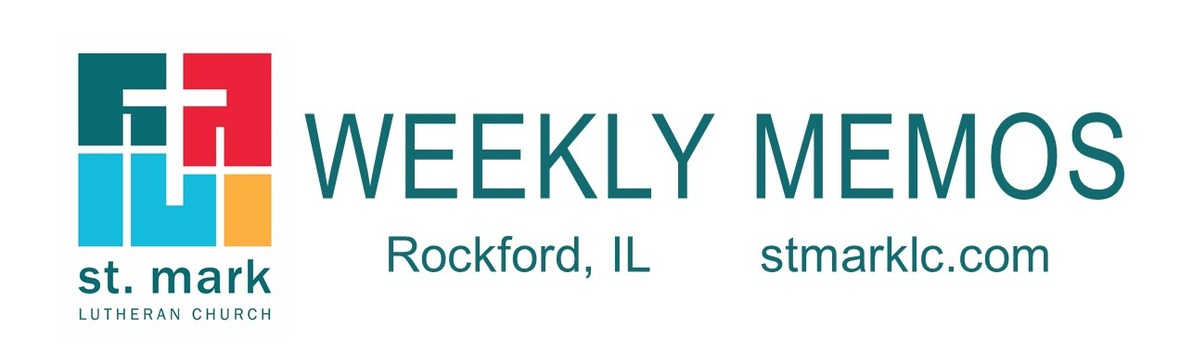table div table+table div table{width:100%;padding:0}table div table+table div table img{width:96.23%;padding:0;float:none}table div table+table div table td{width:100%;padding:0 1.88% 18px}/* styles */## Pastoral Reflection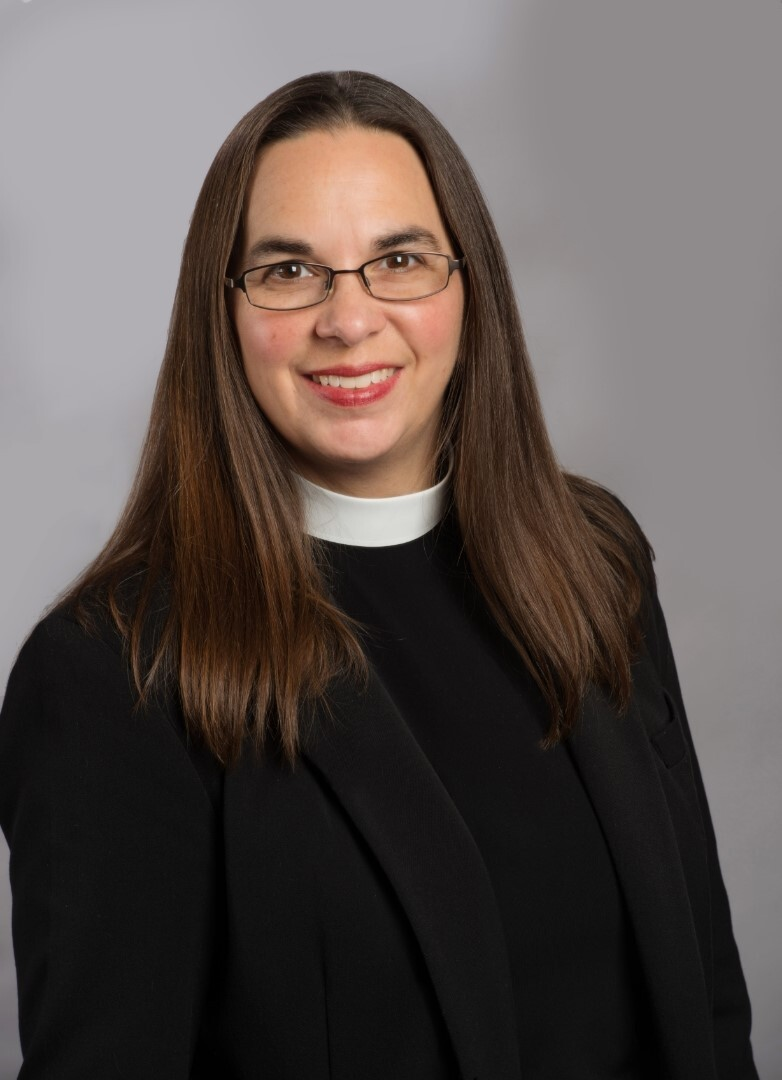Time with God and God's Creation
Pastor Katrina Steingraeber

I love being outside, whether I am working in the yard or just walking around and spending time in nature. It is a great time to connect with God. Read more.

 table div table+table+table+table+table div table{width:100%;padding:0}table div table+table+table+table+table div table img{width:96.23%;padding:0;float:none}table div table+table+table+table+table div table td{width:100%;padding:0 1.88% 18px}/* styles */## Sunday, October 10

9:00 A.M. Indoor Worship Live-Stream in the Sanctuary
10:00 A.M. Fellowship Hour with coffee and donuts
10:10 A.M. Sunday School Begins
11:00 A.M. Worship Outdoors

## Monday, October 11

9:00 A.M. Youth leave to Nachusa Grasslands & Lowden State Park
5:00 P.M. Children's Ministry

## Tuesday, October 12

10:00 A.M. Staff Meeting
11:30 A.M. St. Mark Duplicate Bridge
6:30 P.M. Worship & Music Ministry

## Wednesday, October 13

9:00 A.M. Women's Bible Study
9:00 A.M. Men's Bible Study
9:30 A.M. St. Mark Ringers
12:00 P.M. Miriam Circle at Stockholm Inn
4:30 P.M. Outreach Ministry
6:30 P.M. Confirmation
7:00 P.M. Voices of St. Mark

## Thursday, October 14

9:00 A.M. Cherry Valley Bridge Group
9:30 A.M. Ruth Circle
12:30 P.M. Sew and Sews
6:30 P.M. Emotions Anonymous
6:30 P.M. Blue Lights

## Friday, October 15

7:30 A.M. St. Mark Men's Breakfast at Denny's Restaurant

## Saturday, October 16

10:00 A.M. Visitation for Don Wasson
11:00 A.M. Memorial Service for Don Wasson
12:00 P.M. Luncheon

## Sunday, October 17

9:00 A.M. Indoor Worship Live-Stream in the Sanctuary
10:00 A.M. Fellowship Hour with coffee and donuts
10:10 A.M. Sunday School Begins
10:10 A.M. Learning Hour/ Adult Forum
11:00 A.M. Worship Outdoors

 table div table+table+table+table+table+table+table+table div table{width:100%;padding:0}table div table+table+table+table+table+table+table+table div table img{width:96.23%;padding:0;float:none}table div table+table+table+table+table+table+table+table div table td{width:100%;padding:0 1.88% 18px}/* styles */## News and Events

 table div table+table+table+table+table+table+table+table+table+table div table{width:100%;padding:0}table div table+table+table+table+table+table+table+table+table+table div table img{width:96.23%;padding:0;float:none}table div table+table+table+table+table+table+table+table+table+table div table td{width:100%;padding:0 1.88% 18px}/* styles *//* styles */ Join us for Worship Sunday - 20th Sunday after Pentecost 9:00 am - Traditional Worship in the Sanctuary 10:10 am - Sunday School, Adult Forum and Fellowship Hour with Coffee & Donuts 11:00 am - Blended Worship on the Lawn- Bring your lawn chair or blanket, or you can stay in your car. Our weekly live-stream will occur during the 9:00 am Sanctuary service. Follow this link for the live-stream service Sunday. While the 9:00 am service will be fully traditional, our 11:00 am service outside will have the same musical feel as all our worship this past year, with a blend of traditional and contemporary music. As always, weather-related changes to our outdoor worship will be announced the evening before.
 table div table+table+table+table+table+table+table+table+table+table+table+table div table{width:100%;padding:0}table div table+table+table+table+table+table+table+table+table+table+table+table div table img{width:96.23%;padding:0;float:none}table div table+table+table+table+table+table+table+table+table+table+table+table div table td{width:100%;padding:0 1.88% 18px}/* styles *//* styles */ Sunday morning Adult Forum Our Sunday morning Adult Forum is during the learning hour at 10:10 am. Sunday, we will take a closer look at the Gospel of Mark. Bring your coffee and a donut to the Adult Ministry room for this open discussion led by Pastor Chad. Sunday School and Fellowship also occur during this time between services.
 table div table+table+table+table+table+table+table+table+table+table+table+table+table+table div table{width:100%;padding:0}table div table+table+table+table+table+table+table+table+table+table+table+table+table+table div table img{width:96.23%;padding:0;float:none}table div table+table+table+table+table+table+table+table+table+table+table+table+table+table div table td{width:100%;padding:0 1.88% 18px}/* styles */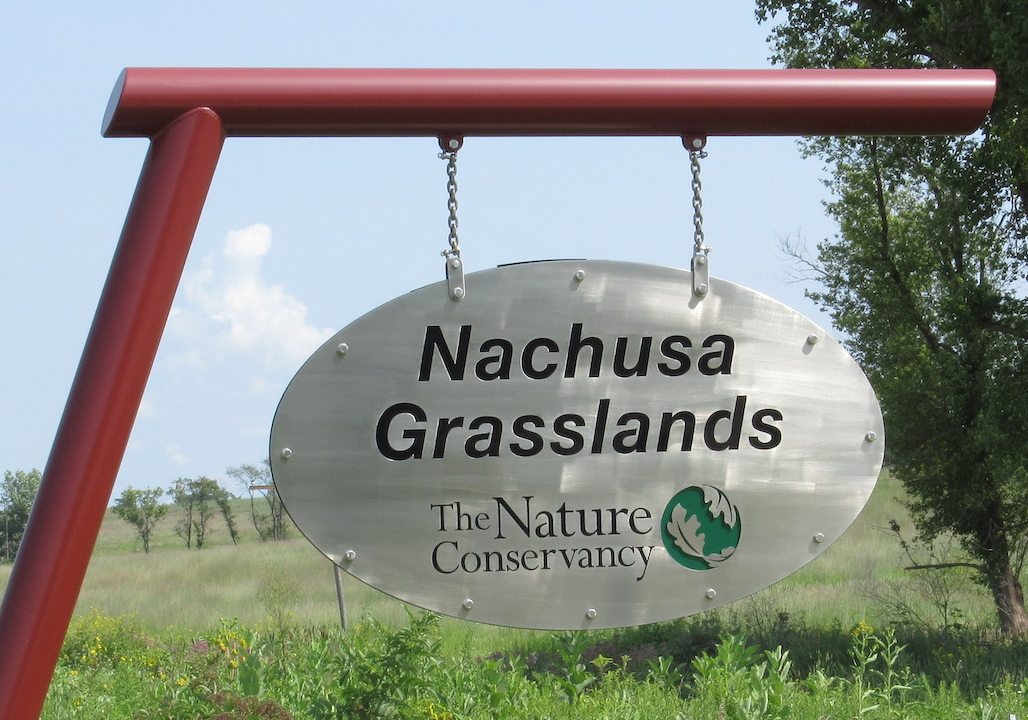Youth Trip to the Nachusa Grasslands and Lowden State Park
On October 11, 2021, for most students there is no school this day, we are planning to take a day trip to the Nachusa Grasslands, in Franklin Grove, Illinois and Lowden State Park, in Oregon, Illinois. We will spend the day exploring God’s beautiful creation. We will learn about habitat restoration at the Nachusa Grasslands and hopefully see bison. We will be hiking at both the Grasslands and Lowden State Park, please wear the appropriate clothing and shoes for the hike. At Lowden, we will visit the recently restored Black Hawk Statue, designed by Lorado Taft as a tribute to Native Americans. We will have opportunities for prayer and conversation, specific to each location. We will pack turkey sandwiches, chips, goldfish crackers, fruit, capri-sun and water for lunches. If you would prefer to pack -your own lunch at home, please do so. If you plan to attend please let Melinda Alekna (815)-871-0390 or Pastor Katrina Steingraeber (815)-988-1215 know, so we can plan accordingly. Any parents/guardians interested in joining us please let us know. This will be a day full of hiking and exploring God’s beautiful creation. We will meet at St. Mark at 9 am and return to St. Mark at 3:30 pm. Masks are required while riding in the cars and inside any buildings.

 table div table+table+table+table+table+table+table+table+table+table+table+table+table+table+table+table div table{width:100%;padding:0}table div table+table+table+table+table+table+table+table+table+table+table+table+table+table+table+table div table img{width:96.23%;padding:0;float:none}table div table+table+table+table+table+table+table+table+table+table+table+table+table+table+table+table div table td{width:100%;padding:0 1.88% 18px}/* styles */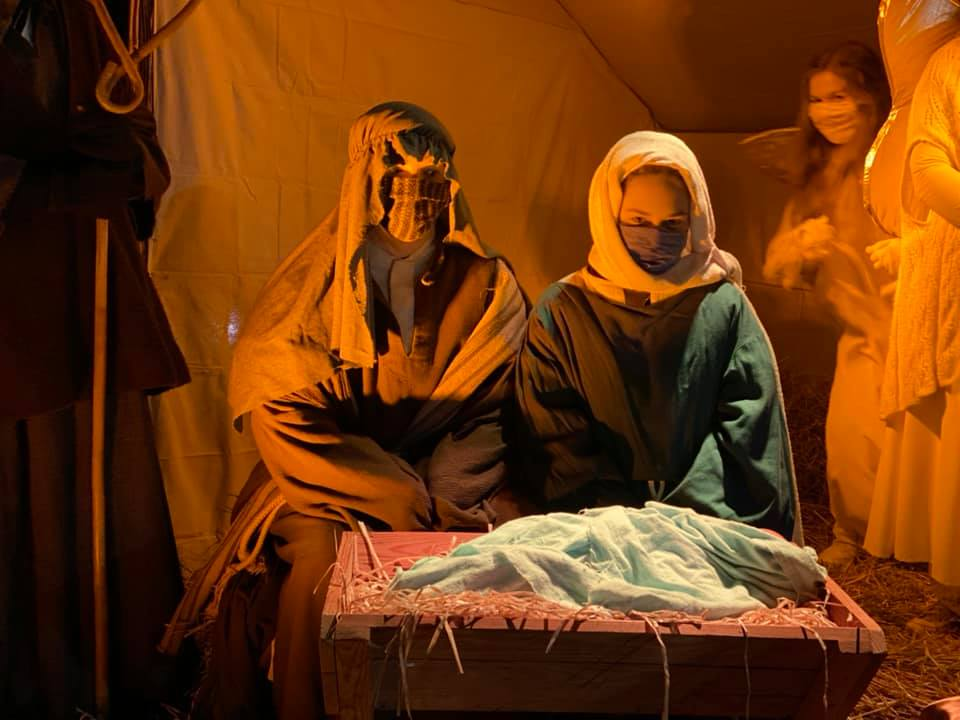Live Nativity Saturday, December 18th Did you attend last year? Would you like to help? More information soon. Please message melinda@stmarklc.com if you are interested in helping. We need set builders, actors, parking lot assistants, cookie makers, etc.

 table div table+table+table+table+table+table+table+table+table+table+table+table+table+table+table+table+table+table div table{width:100%;padding:0}table div table+table+table+table+table+table+table+table+table+table+table+table+table+table+table+table+table+table div table img{width:96.23%;padding:0;float:none}table div table+table+table+table+table+table+table+table+table+table+table+table+table+table+table+table+table+table div table td{width:100%;padding:0 1.88% 18px}/* styles */Family Photo
One of many great things about our Instant Church Directory is that you can send an updated family photo anytime. Some of you have had babies, kids are getting older, and some of our families have grown.

Click on this link to submit your photo. If you want to stop by the church office, we can take the picture for you.

 table div table+table+table+table+table+table+table+table+table+table+table+table+table+table+table+table+table+table+table+table div table{width:100%;padding:0}table div table+table+table+table+table+table+table+table+table+table+table+table+table+table+table+table+table+table+table+table div table img{width:96.23%;padding:0;float:none}table div table+table+table+table+table+table+table+table+table+table+table+table+table+table+table+table+table+table+table+table div table td{width:100%;padding:0 1.88% 18px}/* styles */Affirmation of Baptism/Confirmation is Sunday, October 31st, 2021 at the 9:00 am worship service. We will have a mandatory practice on Wednesday, October 27th at 7:00 pm in the sanctuary, parents/guardians are strongly encouraged to attend with their confirmand. Confirmands please pick out a bible verse and let us know your preferred name for your Confirmation Certificate. Please email Pastor Katrina at prkatrina@stmarklc.com with your preferred name for your Confirmation Certificate as well as your chosen bible verse by October 17th. Thank you! Confirmation is an exciting time for the confirmands, their families, and the church.

 table div table+table+table+table+table+table+table+table+table+table+table+table+table+table+table+table+table+table+table+table+table+table div table{width:100%;padding:0}table div table+table+table+table+table+table+table+table+table+table+table+table+table+table+table+table+table+table+table+table+table+table div table img{width:96.23%;padding:0;float:none}table div table+table+table+table+table+table+table+table+table+table+table+table+table+table+table+table+table+table+table+table+table+table div table td{width:100%;padding:0 1.88% 18px}/* styles */Have you got a pet? Send in your favorite pet picture and we will post on our bulletin board. Please e-mail melinda@stmarklc.com

 table div table+table+table+table+table+table+table+table+table+table+table+table+table+table+table+table+table+table+table+table+table+table+table+table div table{width:100%;padding:0}table div table+table+table+table+table+table+table+table+table+table+table+table+table+table+table+table+table+table+table+table+table+table+table+table div table img{width:96.23%;padding:0;float:none}table div table+table+table+table+table+table+table+table+table+table+table+table+table+table+table+table+table+table+table+table+table+table+table+table div table td{width:100%;padding:0 1.88% 18px}/* styles */October’s St. Mark Celebrates 60 - “Pet Blessings"
We are collecting supplies for Noah’s Ark needs list: bleach, Fabuloso cleaner, washcloths, bath towels, paper towels, HE detergent, dog and cat toys. Monetary donations to benefit wecareforpets.org, ilshorthairrescue.com and friendsofrockfordpolicek9.com You may donate on the St. Mark app, stmarklc.com/give, a check to St. Mark or the individual websites.

 table div table+table+table+table+table+table+table+table+table+table+table+table+table+table+table+table+table+table+table+table+table+table+table+table+table+table div table{width:100%;padding:0}table div table+table+table+table+table+table+table+table+table+table+table+table+table+table+table+table+table+table+table+table+table+table+table+table+table+table div table img{width:96.23%;padding:0;float:none}table div table+table+table+table+table+table+table+table+table+table+table+table+table+table+table+table+table+table+table+table+table+table+table+table+table+table div table td{width:100%;padding:0 1.88% 18px}/* styles */Crop Walk is happening right here at St. Mark! Join us Sunday, October 17th at noon (right after the 11:00 service.) We will start on the east side of the building. Walk with your St. Mark family. We are collecting monetary donations on the church app or you may write a check to St. Mark. We already have a great start to our fundraising from the Outreach committee \$500. Join us to walk and consider donating to this worthy cause. Our goal to match the \$500 and raise a total of \$1000! With faith and prayer we will do it! Donate on the St. Mark ap, stmarklc.com/give or a check to St. Mark.
Will you walk, to end hunger?

 table div table+table+table+table+table+table+table+table+table+table+table+table+table+table+table+table+table+table+table+table+table+table+table+table+table+table+table+table div table{width:100%;padding:0}table div table+table+table+table+table+table+table+table+table+table+table+table+table+table+table+table+table+table+table+table+table+table+table+table+table+table+table+table div table img{width:96.23%;padding:0;float:none}table div table+table+table+table+table+table+table+table+table+table+table+table+table+table+table+table+table+table+table+table+table+table+table+table+table+table+table+table div table td{width:100%;padding:0 1.88% 18px}/* styles */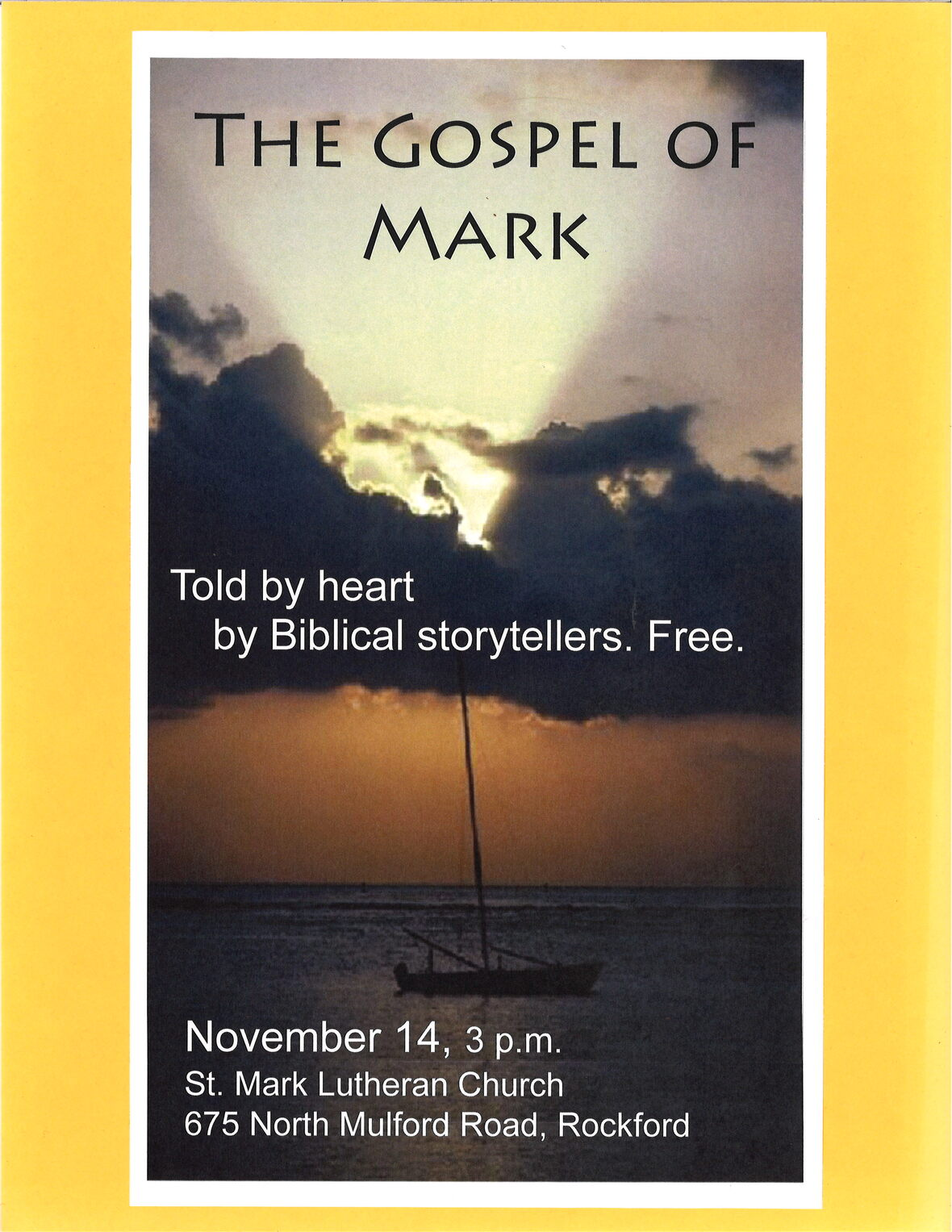/* styles */ Biblical Storytellers present The Gospel of Mark Before the stories of the Bible were composed as written literature, they were told around campfires, dinner tables, and banquet halls as ancient entertainment. On Sunday, November 14 at 3:00 p.m., a team of Biblical storytellers from the Chicago-area Prairie Wind Guild: Tellers of Sacred Stories will present The Gospel of Mark here at St. Mark. As the shortest and most action-packed of the Bible’s four gospels (books about Jesus’ life and ministry), Mark takes about as long as a movie or play. Prairie Wind Guild is associated with the Network of Biblical Storytellers International, which defines the art as “a spiritual discipline…that binds tellers and listeners together in community.” Prairie Wind Guild coordinator Rev. Beth Galbreath says, “Unlike drama with its usual fourth wall, telling is truly a shared experience. The audience can expect to experience Scripture, in this case, Mark’s story of Jesus, coming to life through the telling.” Admission is free, though donations to defray expenses will be gratefully accepted.
 table div table+table+table+table+table+table+table+table+table+table+table+table+table+table+table+table+table+table+table+table+table+table+table+table+table+table+table+table+table+table+table div table{width:100%;padding:0}table div table+table+table+table+table+table+table+table+table+table+table+table+table+table+table+table+table+table+table+table+table+table+table+table+table+table+table+table+table+table+table div table img{width:96.23%;padding:0;float:none}table div table+table+table+table+table+table+table+table+table+table+table+table+table+table+table+table+table+table+table+table+table+table+table+table+table+table+table+table+table+table+table div table td{width:100%;padding:0 1.88% 18px}/* styles */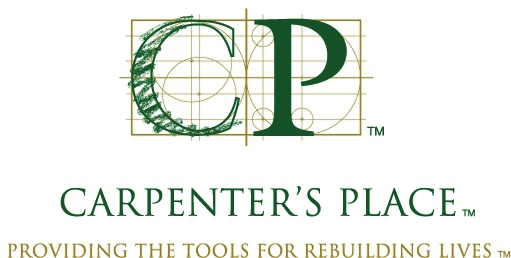Carpenter's Place - Urgent Help this October
Click on this link to find out ways to help.

 table div table+table+table+table+table+table+table+table+table+table+table+table+table+table+table+table+table+table+table+table+table+table+table+table+table+table+table+table+table+table+table+table+table div table{width:100%;padding:0}table div table+table+table+table+table+table+table+table+table+table+table+table+table+table+table+table+table+table+table+table+table+table+table+table+table+table+table+table+table+table+table+table+table div table img{width:96.23%;padding:0;float:none}table div table+table+table+table+table+table+table+table+table+table+table+table+table+table+table+table+table+table+table+table+table+table+table+table+table+table+table+table+table+table+table+table+table div table td{width:100%;padding:0 1.88% 18px}/* styles */Save the Date Sunday, March 6, for St. Mark Talent Show.
We want you to participate and enjoy an afternoon of talent, fun, laughter with your St. Mark family. This will be a youth fundraiser for the Gathering 2022. Watch for more details.

 table div table+table+table+table+table+table+table+table+table+table+table+table+table+table+table+table+table+table+table+table+table+table+table+table+table+table+table+table+table+table+table+table+table+table+table div table{width:100%;padding:0}table div table+table+table+table+table+table+table+table+table+table+table+table+table+table+table+table+table+table+table+table+table+table+table+table+table+table+table+table+table+table+table+table+table+table+table div table img{width:96.23%;padding:0;float:none}table div table+table+table+table+table+table+table+table+table+table+table+table+table+table+table+table+table+table+table+table+table+table+table+table+table+table+table+table+table+table+table+table+table+table+table div table td{width:100%;padding:0 1.88% 18px}/* styles */The Wednesday morning Women's Bible Study
We meet regularly on Wednesday morning at 9:00 in the Conference Room. We will begin with a study of Galatians. Paul's letter to the Galatians is one argument after another about the amazing grace we receive from God. The Bible Study is open to all the women of St. Mark and friends. The Bible Study is based on Scripture, discussion, and life experiences from the participants. If you would like more information call Deb Iverson.The Wednesday morning Men's Bible Study meets at 9:00 a.m. in the cafe. All men are welcome.

 table div table+table+table+table+table+table+table+table+table+table+table+table+table+table+table+table+table+table+table+table+table+table+table+table+table+table+table+table+table+table+table+table+table+table+table+table+table+table div table{width:100%;padding:0}table div table+table+table+table+table+table+table+table+table+table+table+table+table+table+table+table+table+table+table+table+table+table+table+table+table+table+table+table+table+table+table+table+table+table+table+table+table+table div table img{width:96.23%;padding:0;float:none}table div table+table+table+table+table+table+table+table+table+table+table+table+table+table+table+table+table+table+table+table+table+table+table+table+table+table+table+table+table+table+table+table+table+table+table+table+table+table div table td{width:100%;padding:0 1.88% 18px}/* styles */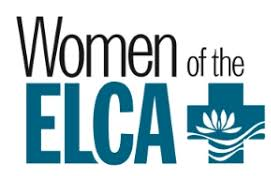Miriam Circle will meet for lunch at 12 Noon on Wednesday, October 13 at Stockholm Inn. Our quest will be John Hargrove and he will share information about Habitat for Humanity mission and Restore. We welcome all women to join the Miriam Circle on the second Wednesday of each month. Please RSVP to Betty Sparks: Ph. 815 636-1122, email kbsp4968@msn.com

Ruth Circle will meet on Thursday, October 14th, at 9:30 in Fellowship Hall. Our meetings are open for all members of the church. Please consider joining our group for fellowship and friendship. Please RSVP to Judy Penticoff. teachem4@comcast.net
(815) 985-3427.

 table div table+table+table+table+table+table+table+table+table+table+table+table+table+table+table+table+table+table+table+table+table+table+table+table+table+table+table+table+table+table+table+table+table+table+table+table+table+table+table+table div table{width:100%;padding:0}table div table+table+table+table+table+table+table+table+table+table+table+table+table+table+table+table+table+table+table+table+table+table+table+table+table+table+table+table+table+table+table+table+table+table+table+table+table+table+table+table div table img{width:96.23%;padding:0;float:none}table div table+table+table+table+table+table+table+table+table+table+table+table+table+table+table+table+table+table+table+table+table+table+table+table+table+table+table+table+table+table+table+table+table+table+table+table+table+table+table+table div table td{width:100%;padding:0 1.88% 18px}/* styles */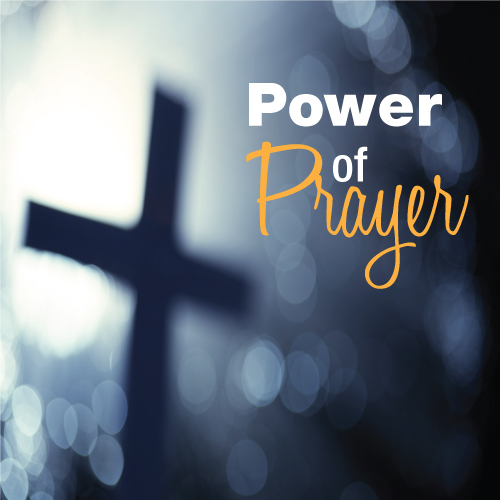St. Mark Prayer Chain
Would you like to be a part of the St. Mark prayer chain? Prayer requests come into the church office weekly. As we receive prayer requests we would send out an e-mail and ask that you pray daily for the persons mentioned in the requests. All requests are to remain confidential. Please e-mail melinda@stmarklc.com if you would like to participate in the prayer chain. “Be anxious for nothing, but in everything by prayer and supplication, with thanksgiving, let your requests be made known to God.” Colossians 4:2.

 table div table+table+table+table+table+table+table+table+table+table+table+table+table+table+table+table+table+table+table+table+table+table+table+table+table+table+table+table+table+table+table+table+table+table+table+table+table+table+table+table+table+table div table{width:100%;padding:0}table div table+table+table+table+table+table+table+table+table+table+table+table+table+table+table+table+table+table+table+table+table+table+table+table+table+table+table+table+table+table+table+table+table+table+table+table+table+table+table+table+table+table div table img{width:96.23%;padding:0;float:none}table div table+table+table+table+table+table+table+table+table+table+table+table+table+table+table+table+table+table+table+table+table+table+table+table+table+table+table+table+table+table+table+table+table+table+table+table+table+table+table+table+table+table div table td{width:100%;padding:0 1.88% 18px}/* styles */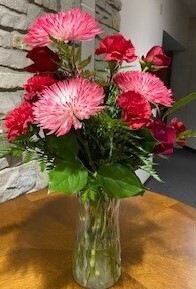Sign up for Altar Flowers There are some dates still available to sponsor a flower arrangement on Sundays. This can be in honor or memory of someone or just because. The price for one bouquet is \$35. To sign up for flowers you can call the office at 815-398-3557, or click on this link. If you sign up online, put your memory or honor of in the comment line and pay here or write a check to St. Mark. If you have the mobile app you can do all of it on there.
You may take your flowers home after the service or stop by the office during the week to pick them up.

 table div table+table+table+table+table+table+table+table+table+table+table+table+table+table+table+table+table+table+table+table+table+table+table+table+table+table+table+table+table+table+table+table+table+table+table+table+table+table+table+table+table+table+table+table div table{width:100%;padding:0}table div table+table+table+table+table+table+table+table+table+table+table+table+table+table+table+table+table+table+table+table+table+table+table+table+table+table+table+table+table+table+table+table+table+table+table+table+table+table+table+table+table+table+table+table div table img{width:96.23%;padding:0;float:none}table div table+table+table+table+table+table+table+table+table+table+table+table+table+table+table+table+table+table+table+table+table+table+table+table+table+table+table+table+table+table+table+table+table+table+table+table+table+table+table+table+table+table+table+table div table td{width:100%;padding:0 1.88% 18px}/* styles */## Youth Ministry

 table div table+table+table+table+table+table+table+table+table+table+table+table+table+table+table+table+table+table+table+table+table+table+table+table+table+table+table+table+table+table+table+table+table+table+table+table+table+table+table+table+table+table+table+table+table+table div table{width:100%;padding:0}table div table+table+table+table+table+table+table+table+table+table+table+table+table+table+table+table+table+table+table+table+table+table+table+table+table+table+table+table+table+table+table+table+table+table+table+table+table+table+table+table+table+table+table+table+table+table div table img{width:96.23%;padding:0;float:none}table div table+table+table+table+table+table+table+table+table+table+table+table+table+table+table+table+table+table+table+table+table+table+table+table+table+table+table+table+table+table+table+table+table+table+table+table+table+table+table+table+table+table+table+table+table+table div table td{width:100%;padding:0 1.88% 18px}/* styles */Trunk or Treat!
Middle and High School Youth Sunday, October 24th, we are helping at a trunk or treat for Mosaic! It will be great fun! Let Melinda Alekna know you are interested. Need more information? 815-871-0390 or Melinda@stmarklc.comELCA Youth Gathering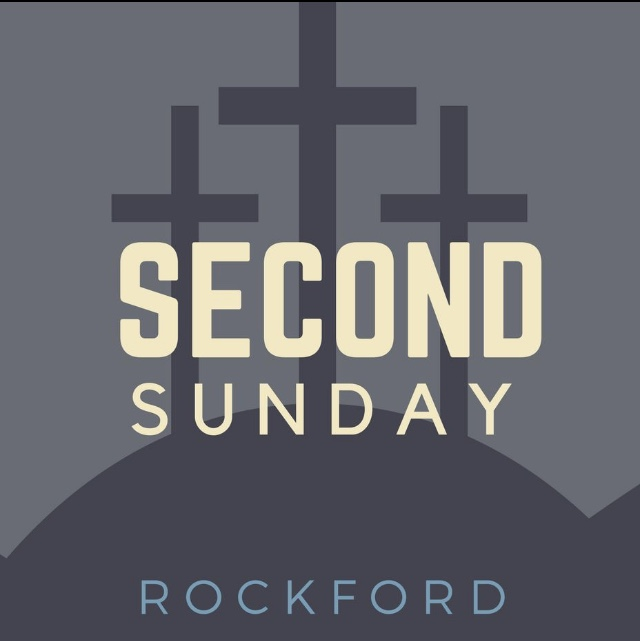Second Sunday is happening this Sunday, October 10, 6:30-8:00 P.M. Youth in 8th grade through high school are invited to join us at St. Mark. There will be a campfire, games, and s’mores! We are hosting and plan to have youth from other churches joining us. Bring a friend and be here! Each Second Sunday we will visit other churches for Second Sunday. November(Christ Lutheran)

 table div table+table+table+table+table+table+table+table+table+table+table+table+table+table+table+table+table+table+table+table+table+table+table+table+table+table+table+table+table+table+table+table+table+table+table+table+table+table+table+table+table+table+table+table+table+table+table+table+table+table div table{width:100%;padding:0}table div table+table+table+table+table+table+table+table+table+table+table+table+table+table+table+table+table+table+table+table+table+table+table+table+table+table+table+table+table+table+table+table+table+table+table+table+table+table+table+table+table+table+table+table+table+table+table+table+table+table div table img{width:96.23%;padding:0;float:none}table div table+table+table+table+table+table+table+table+table+table+table+table+table+table+table+table+table+table+table+table+table+table+table+table+table+table+table+table+table+table+table+table+table+table+table+table+table+table+table+table+table+table+table+table+table+table+table+table+table+table div table td{width:100%;padding:0 1.88% 18px}/* styles */## Children's MinistrySunday School
Welcome back to Sunday school – in person! Hooray!

Sunday school will meet weekly from 10:10-10:50 a.m. Families are encouraged to worship together at either the 9:00 or 11:00 service.

Following guidance for children’s settings here in Illinois, all children and adults are required to wear masks in the Sunday school space regardless of vaccination status. As the situation changes, we will adjust as well.

Register here and get excited for Sunday school! And as always, contact julie@stmarklc.com for more details.

 table div table+table+table+table+table+table+table+table+table+table+table+table+table+table+table+table+table+table+table+table+table+table+table+table+table+table+table+table+table+table+table+table+table+table+table+table+table+table+table+table+table+table+table+table+table+table+table+table+table+table+table+table+table div table{width:100%;padding:0}table div table+table+table+table+table+table+table+table+table+table+table+table+table+table+table+table+table+table+table+table+table+table+table+table+table+table+table+table+table+table+table+table+table+table+table+table+table+table+table+table+table+table+table+table+table+table+table+table+table+table+table+table+table div table img{width:96.23%;padding:0;float:none}table div table+table+table+table+table+table+table+table+table+table+table+table+table+table+table+table+table+table+table+table+table+table+table+table+table+table+table+table+table+table+table+table+table+table+table+table+table+table+table+table+table+table+table+table+table+table+table+table+table+table+table+table+table div table td{width:100%;padding:0 1.88% 18px}/* styles */## Our St. Mark members:

Ben Granados, John Lund, Dennis Clark, Linda Vivian, Jack Sowl, Lorine Kortus, Jan Cotter, Roger Lind, Diane Swanson, Sherri Lindquist, Bette Patterson, Keith Haggestad.

## Family and friends of St. Mark members:

Katelynn Audette, Mike Glynn, Shirley Brown,
Ron Ganschow, Bill Ganschow, Clyde Webster, Bernice, Conrad Semmelroth, Nici King, Debbie Adams, Linda Johnson, Eva Love Sherbondy, Norma Comstock, Carolyn King.

## Those serving in the military and their families:

Hunter Haggestad, Tristan Davenport, Scot Hornick,
Bailey Panjkovich, Jim Tammen, Brandon Ballenger, Taylor Eversole, Dean Barron.

Strengthen and encourage those in public health services and in the medical profession: care-givers, nurses, attendants, doctors, all who commit themselves to care for the sick and their families.

 table div table+table+table+table+table+table+table+table+table+table+table+table+table+table+table+table+table+table+table+table+table+table+table+table+table+table+table+table+table+table+table+table+table+table+table+table+table+table+table+table+table+table+table+table+table+table+table+table+table+table+table+table+table+table+table+table div table{width:100%;padding:0}table div table+table+table+table+table+table+table+table+table+table+table+table+table+table+table+table+table+table+table+table+table+table+table+table+table+table+table+table+table+table+table+table+table+table+table+table+table+table+table+table+table+table+table+table+table+table+table+table+table+table+table+table+table+table+table+table div table img{width:96.23%;padding:0;float:none}table div table+table+table+table+table+table+table+table+table+table+table+table+table+table+table+table+table+table+table+table+table+table+table+table+table+table+table+table+table+table+table+table+table+table+table+table+table+table+table+table+table+table+table+table+table+table+table+table+table+table+table+table+table+table+table+table div table td{width:100%;padding:0 1.88% 18px}/* styles *//* styles */ Office Hours Monday through Friday 9:00 A.M. - 4:00 P.M. Telephone: 815-398-3557 Publications St. Mark Memos, the weekly e-newsletter, is distributed each Thursday. The deadline for submission is Monday by 3:00 P.M. Please click to send submissions. Worship Services Miss a Sunday at St. Mark? Please visit our website and watch the service online. Please click to watch.
 table div table+table+table+table+table+table+table+table+table+table+table+table+table+table+table+table+table+table+table+table+table+table+table+table+table+table+table+table+table+table+table+table+table+table+table+table+table+table+table+table+table+table+table+table+table+table+table+table+table+table+table+table+table+table+table+table+table+table div table{width:100%;padding:0}table div table+table+table+table+table+table+table+table+table+table+table+table+table+table+table+table+table+table+table+table+table+table+table+table+table+table+table+table+table+table+table+table+table+table+table+table+table+table+table+table+table+table+table+table+table+table+table+table+table+table+table+table+table+table+table+table+table+table div table img{width:96.23%;padding:0;float:none}table div table+table+table+table+table+table+table+table+table+table+table+table+table+table+table+table+table+table+table+table+table+table+table+table+table+table+table+table+table+table+table+table+table+table+table+table+table+table+table+table+table+table+table+table+table+table+table+table+table+table+table+table+table+table+table+table+table+table div table td{width:100%;padding:0 1.88% 18px}/* styles */